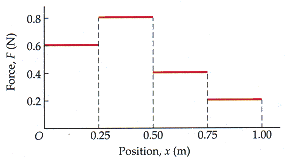# Solving for final position with a negative work value

• Selayna

## Homework Statement

An object is acted on by the force shown in the picture shown below:What is the final position of the object if its initial position is x = 0.50 m and the work done on it is equal to -0.29 J?

Initial position = Xi = 0.5 m
Work = W = -0.29 J
Force = F
displacement = d
Final position = Xf = ? m

## Homework Equations

W = F(d - Xi) + F(d-d) + F(Xf - d)
W = Fd

## The Attempt at a Solution

[/B]
I worked on the first part of this problem, where work was equal to 0.12 J and the initial position was 0.5 m, using this equation:
0.12 = 0.8(0.5 - 0.5) + 0.4(0.75-0.5) + 0.2(Xf - 0.75)

I assumed, since W = Fd, and displacement can't have a negative value, Force would have to have a negative value to get a negative value for Work. Using this logic, I modified the original equation to use a negative Force:
W = -F(d - Xi) - F(d-d) - F(Xf - d)

Using this equation, I went through these steps:
1. Plug in values
-0.29 = -0.8(0.5 - 0.5) - 0.4(0.75-0.5) - 0.2(Xf - 0.75)
2. Reduce
-0.29 = 0 - 0.1 + 0.15 - 0.2Xf
3. Subtract 0.15 and add 0.1 to both sides
-0.34 = -0.2Xf
4. Divide both sides by 0.2
1.7 = Xf

However, the answer I got for the final position (1.7), was incorrect. I'm assuming my initial logic using negative values for force was incorrect, but I'm not sure how else I would solve the problem with a negative work value.

Any help is appreciated. Thanks!

Last edited:

Hello, and welcome to PF!

The force values are all positive as indicated in the graph. This means that the force is always directed toward the positive x direction.

You mentioned that you thought that displacement cannot be negative. But this is not true. You may assume that the object can move in either direction along the x axis. For example, the object might have been given an initial velocity in the negative x direction so that it will move toward negative x even though there is a force acting toward positive x. (Similar to throwing a ball upward. The ball travels upward while the force of gravity acts downward.)

If a force acts in the positive x direction while an object moves toward the negative x direction (i.e., negative displacement), what is the sign of the work?

Think about how the object can start at x = 0.50 m and move such that the work done on the object is negative.

Last edited:
displacement can't have a negative value
It starts at 0.5m. If at some later time its position is < 0.5m then that's a negative displacement.
The only way I can make sense of the question is that there are other forces acting on the object, and these can overcome the force F to result in the object's displacement being opposite to F.